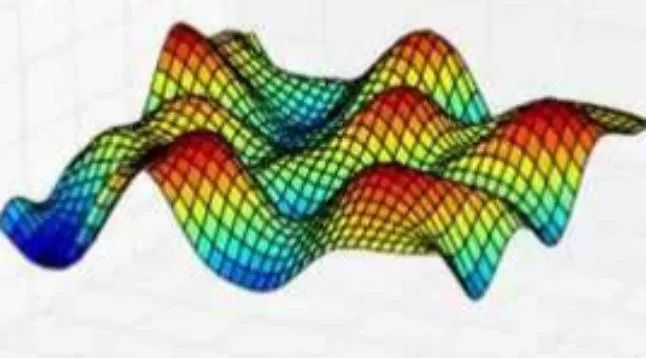## 图文详解高斯过程（一）——含代码

2017 年 12 月 18 日 论智 Alex Bridgland## 什么是高斯过程？

• 假设有一个隐藏函数：f：ℝ→ℝ，我们要对它建模；

• fx

## 用高斯建模函数

GP背后的关键思想是可以使用无限维多变量高斯分布来对函数进行建模。换句话说，输入空间中的每个点都与一个随机变量相关联，而它们的联合分布可以被作为多元高斯分布建模。

```

`def plot_unit_gaussian_samples(D):`

`    p = figure(plot_width=800, plot_height=500,`

`               title='Samples from a unit {}D Gaussian'.format(D))`

`    xs = np.linspace(0, 1, D)`

`    for color in Category10:`

`        ys = np.random.multivariate_normal(np.zeros(D), np.eye(D))`

`        p.line(xs, ys, line_width=1, color=color)`

`    return p`

`show(plot_unit_gaussian_samples(2))`

```

```

`show(plot_unit_gaussian_samples(20))`

```

## 用核函数实现平滑

```

`def k(xs, ys, sigma=1, l=1):`

`    """Sqared Exponential kernel as above but designed to return the whole`

`    covariance matrix - i.e. the pairwise covariance of the vectors xs & ys.`

`    Also with two parameters which are discussed at the end."""`

`    # Pairwise difference matrix.`

`    dx = np.expand_dims(xs, 1) - np.expand_dims(ys, 0)`

`    return (sigma ** 2) * np.exp(-((dx / l) ** 2) / 2)`

`def m(x):`

`    """The mean function. As discussed, we can let the mean always be zero."""`

`    return np.zeros_like(x)`

```

```

`N = 100`

`x = np.linspace(-2, 2, N)`

`y = np.linspace(-2, 2, N)`

`d = k(x, y)`

`color_mapper = LinearColorMapper(palette="Plasma256", low=0, high=1)`

`p = figure(plot_width=400, plot_height=400, x_range=(-2, 2), y_range=(-2, 2),`

`           title='Visualisation of k(x, x\')', x_axis_label='x',`

`           y_axis_label='x\'', toolbar_location=None)`

`p.image(image=[d], color_mapper=color_mapper, x=-2, y=-2, dw=4, dh=4)`

`color_bar = ColorBar(color_mapper=color_mapper, ticker=BasicTicker(),`

`                     label_standoff=12, border_line_color=None, location=(0,0))`

`p.add_layout(color_bar, 'right')`

`show(p)`

```

```

`p = figure(plot_width=800, plot_height=500)`

`D = 20`

`xs = np.linspace(0, 1, D)`

`for color in Category10:`

`    ys = np.random.multivariate_normal(m(xs), k(xs, xs))`

`    p.circle(xs, ys, size=3, color=color)`

`    p.line(xs, ys, line_width=1, color=color)`

`show(p)`

```

```

`n = 100`

`xs = np.linspace(-5, 5, n)`

`K = k(xs, xs)`

`mu = m(xs)`

`p = figure(plot_width=800, plot_height=500)`

`for color in Category10:`

`    ys = np.random.multivariate_normal(mu, K)`

`    p.line(xs, ys, line_width=2, color=color)`

`show(p)`

```

## 用先验和观察进行预测

```

`# coefs[i] is the coefficient of x^i`

`coefs = [6, -2.5, -2.4, -0.1, 0.2, 0.03]`

`def f(x):`

`    total = 0`

`    for exp, coef in enumerate(coefs):`

`        total += coef * (x ** exp)`

`    return total`

`xs = np.linspace(-5.0, 3.5, 100)`

`ys = f(xs)`

`p = figure(plot_width=800, plot_height=400, x_axis_label='x',`

`           y_axis_label='f(x)', title='The hidden function f(x)')`

`p.line(xs, ys, line_width=2)`

`show(p)`

```

K=κ(x,x)，均值函数m(x)=0。

```

`x_obs = np.array([-4, -1.5, 0, 1.5, 2.5, 2.7])`

`y_obs = f(x_obs)`

`x_s = np.linspace(-8, 7, 80)`

```

```

`K = k(x_obs, x_obs)`

`K_s = k(x_obs, x_s)`

`K_ss = k(x_s, x_s)`

`K_sTKinv = np.matmul(K_s.T, np.linalg.pinv(K))`

`mu_s = m(x_s) + np.matmul(K_sTKinv, y_obs - m(x_obs))`

`Sigma_s = K_ss - np.matmul(K_sTKinv, K_s)`

```

```

`p = figure(plot_width=800, plot_height=600, y_range=(-7, 8))`

`y_true = f(x_s)`

`p.line(x_s, y_true, line_width=3, color='black', alpha=0.4,`

`       line_dash='dashed', legend='True f(x)')`

`p.cross(x_obs, y_obs, size=20, legend='Training data')`

`stds = np.sqrt(Sigma_s.diagonal())`

`err_xs = np.concatenate((x_s, np.flip(x_s, 0)))`

`err_ys = np.concatenate((mu_s + 2 * stds, np.flip(mu_s - 2 * stds, 0)))`

`p.patch(err_xs, err_ys, alpha=0.2, line_width=0, color='grey',`

`        legend='Uncertainty')`

`for color in Category10:`

`    y_s = np.random.multivariate_normal(mu_s, Sigma_s)`

`    p.line(x_s, y_s, line_width=1, color=color)`

`p.line(x_s, mu_s, line_width=3, color='blue', alpha=0.4, legend='Mean')`

`show(p)`

```

## 下篇预告

Github：gist.github.com/Bridgo/429594942ff51037ecc703905e40c56262

### 相关内容017007001000800280081071011300100060

17+阅读 · 2019年10月18日
AI研习社
7+阅读 · 2019年3月1日

10+阅读 · 2019年2月13日

8+阅读 · 2019年2月11日

8+阅读 · 2018年7月8日

7+阅读 · 2018年5月10日

10+阅读 · 2018年1月18日

35+阅读 · 2020年7月4日

55+阅读 · 2020年6月6日

163+阅读 · 2020年6月3日

112+阅读 · 2020年6月3日

63+阅读 · 2020年5月9日

130+阅读 · 2020年5月2日

13+阅读 · 2020年4月20日

88+阅读 · 2020年3月15日

Pasquale Minervini,Matko Bošnjak,Tim Rocktäschel,Sebastian Riedel,Edward Grefenstette
10+阅读 · 2019年12月17日
7+阅读 · 2019年9月10日
Jingzhao Zhang,Lu Mi,Macheng Shen
6+阅读 · 2018年12月13日
Yen-Yu Chang,Fan-Yun Sun,Yueh-Hua Wu,Shou-De Lin
11+阅读 · 2018年9月6日
Badri N. Patro,Vinod K. Kurmi,Sandeep Kumar,Vinay P. Namboodiri
6+阅读 · 2018年6月15日
Ziwei Zhang,Peng Cui,Haoyang Li,Xiao Wang,Wenwu Zhu
4+阅读 · 2018年5月7日
Honggang Zhou,Yunchun Li,Hailong Yang,Wei Li,Jie Jia
3+阅读 · 2018年4月26日
Ermo Wei,Drew Wicke,David Freelan,Sean Luke
10+阅读 · 2018年4月25日
Ngoc-Trung Tran,Tuan-Anh Bui,Ngai-Man Cheung
10+阅读 · 2018年3月23日
Liqian Ma,Qianru Sun,Stamatios Georgoulis,Luc Van Gool,Bernt Schiele,Mario Fritz
6+阅读 · 2018年1月21日
Top